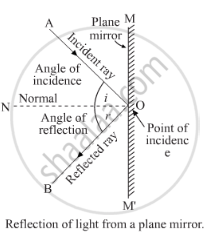Share

# State and Explain the Laws of Reflection of Light at a Plane Surface (Like a Plane Mirror), with the Help of a Labelled Ray-diagram. Mark the Angles of 'Incidence' and 'Reflection' Clearly on the D - CBSE Class 10 - Science

ConceptLight Reflection and Refraction Introduction

#### Question

State and explain the laws of reflection of light at a plane surface (like a plane mirror), With the help of a labelled ray-diagram. Mark the angles of 'incidence' and 'reflection' clearly on the diagram. If the angle of reflection is 47.5°, what will be the angle of incidence?

#### Solutiona) The two laws of reflection are:

1. The angle of incidence is always equal to the angle of reflection . If the angle of incidence is i and the angle of reflection is r, then, according to the first law of reflection,   .∠i=∠r
For example, if one measures the angle of reflection
1. The second law of reflection states that the incident ray, the reflected ray and the normal (at the point of incidence), all lie in the same plane. For example, in the figure, the incident ray AO, the reflected ray OB and the normal ON, all lie in the same plane.

(b) If the angle of reflection is 47.5°, the angle of incidence will also be 47.5° in accordance with the first law of reflection, which states that the angle of reflection is always equal to the angle of incidence.

Is there an error in this question or solution?

#### APPEARS IN

Solution State and Explain the Laws of Reflection of Light at a Plane Surface (Like a Plane Mirror), with the Help of a Labelled Ray-diagram. Mark the Angles of 'Incidence' and 'Reflection' Clearly on the D Concept: Light Reflection and Refraction Introduction.
S